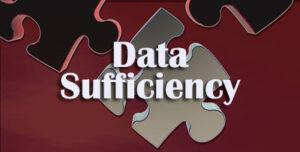# MAT Data Sufficiency Questions and Answers for MBA Entrance Test

MAT Data Sufficiency Papers are available on this page. Check Management Aptitude Test Data Sufficiency Papers here. Aspirants can get MAT Data Sufficiency Model Papers on our site. Applicants can find Management Aptitude Test Data Sufficiency Papers on our site.Candidates who are in search for MAT Data Sufficiency Model Papers can get them here. We provided valuable information regarding MAT Data Sufficiency Papers for Exam. So, interested people can use these Data Sufficiency Sample Papers in their preparation.

All these MAT Data Sufficiency question papers are collected from Previous Year Question Papers. So, these questions are only for practice purpose. Don’t think these questions will be asked in the exam. Aspirants who are going to appear for MAT examination can find the data sufficiency exam pattern details below.

## Model Questions on Data Sufficiency for MAT

Directions: Each of the questions below consists of a question and two statements numbered I and II given below it. You have to decide whether the data provided in the statements are sufficient to answer the question. Read both the statements and give answer
(1) if the data in statement I alone are sufficient to answer the question, while the data in statement II alone are not sufficient to answer the question;
(2) if the data in statement II alone are sufficient to answer the question, while the data in statement I alone are not sufficient to answer the question
(3) if the data either in statement I alone or in statement II alone are sufficient to answer the question;
(4) if the data given in both the statements I and II together are not sufficient to answer the question
(5) If the data in both the statements I and II together are necessary to answer the question.

1. What is the position of K from the right end in a row?
I. There are even numbers of students in the row. There are not more than 11 students in the row.
II. C stands at the extreme left end of the line. There are five students between C and B. O is third lo the right.

2. In general body election, 3 candidates p, q and r were contesting for a membership of the board. How many votes did each receive?
II. Total votes cast were 703

3. If C1 and C2 are the circumferences of the outer and inner circles respectively. What is C1 : C2?
I. The two circles are concentric
II. ΔABC and ΔPQR are right angle Triangles

4. Salary of A and B is in ratio 3 :4 and expenditure is in ratio 4:5. What is the ratio of their saving?
I. B’s saving is 25% of his salary.
II. B’s salary is Rs 2500.

5. What is the middle number of 7 consecutive whole numbers?
I. Product of number is 702800
II. Sum of the numbers is 105

6. How many ice cubes can be accommodated in a container?
I. The length and breadth of the container is 20 cm and15 cm, respectively
II. The edge of the ice cube is 2 cm

7. Ram got Rs 1500 as dividend form a company. What is the rate of interest given by the company?
I. The dividend paid last year was 10%
II. Ram has 350 shares of Rs 10 denomination

8. A man distributed 43 chocolates to his children How many of his children are more than five years old?
I. A child older than five years gets 5 chocolates.
II. A child 5 years or younger in age gets 6 chocolates.

9. A train running at a certain speed crosses a platform in 30 secs. What is the speed of the train?
I. Length of the train is 240 m.
II. The train crosses a man who is on the platform in 12 seconds.

10. The weights of A, B and C are in the ratio of 8:7:5. A’s weight is 60% more than C’s. Find the weight of B.
I. Total weight of A, B and C is 300 kg.
II. Difference between A’s and C’s weight is 45 kg.

## Read More Questions on Data Sufficiency

11. A shopkeeper sells some articles, taking profit of 20% on the cost price. Find the amount of profit.
I. Cost price of the article is Rs 2400.
II. Selling price of the article is Rs 480 more than the cost price.

12. A 540m long plot of rectangular land is to be fenced. Find the cost of fencing per square metre.
I. Breadth of the rectangular plot is 60 m.
II. Length of the rectangular plot is 120 m.

13. The difference between the simple interest and the compound interest on a sum of money at the end of four years is Rs 1282. Find the sum.
I. Amount of simple interest accrued after four years is Rs 4000.
II. Rate of interest is 10% per annum

14. A line graph on a graph sheet shows the revenue for each year from 1990 through 1998 by points and joins the successive points by straight line segments. The point for revenue of 1990 is labeled A, that for 1991 as B, and that for 1992 as C. What is the ratio of growth in revenue between 1991-92 and 1990-91?
I. The angle between AB and X-axis when measured with a protractor is 40 degrees, and the angle between CB and x-axis is 80 degrees.
II. The scale of y-axis is 1 cm = Rs 1000

15. There is a circle with centre C at the origin and radius r cm. Two tangents arc drawn from an external point D at a distance d cm from the centre. What are the angles between each tangent and the X-axis?
I. The co-ordinates of D are given.
II. The X-axis bisects one of the tangents.